### Home > CALC > Chapter 1 > Lesson 1.2.1 > Problem1-20

1-20.
1. Let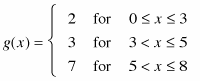1-20 HW eTool (Desmos) Homework Help ✎

1. Sketch the graph of g(x). Is this function continuous? Explain.

2. Shade the area between g(x) and the x-axis. Find A(g, 0 ≤ x ≤ 8).

3. The equation g(x) is an example of a step function. Why do you think it is called a step function?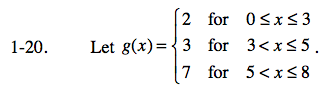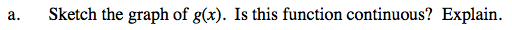Continuous functions must have connected pieces. There can be no jumps or holes. What happens to g(x) at x = 3 and at x = 5?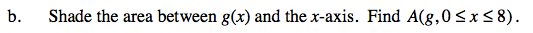Area of Rectangle A: 2 × 3 = 6 units2
Area of Rectangle B: 3 × 2 = 6 units2
Area of Rectangle C: 7 × 3 = 21 units2

Rectangle A + Rectangle B + Rectangle C = 33 units2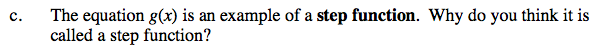The shape of the graph should help answer this question.

Use the eTool below to examine the graph of g(x).
Click the link at right for the full version of the eTool: Calc 1-20 HW eTool